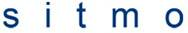# Algebreic expressioni can take this down to## The Attempt at a Solution

So you are trying to simplify the expression, I take it?

$${(\sqrt{a} + 1)^2 - {a-\sqrt {ax} \over \sqrt a - \sqrt x} \over (\sqrt a + 1)^3 - a \sqrt a + 2}$$

= $${(a + 2 \sqrt a + 1)(\sqrt a - \sqrt x) - (a - \sqrt {ax}) \over (\sqrt a - \sqrt x)((\sqrt a + 1)^3 - a \sqrt a + 2)}$$

= $${a \sqrt a - a \sqrt x +2a - 2 \sqrt a \sqrt x + \sqrt a - \sqrt x - a + \sqrt{ax} \over (\sqrt a - \sqrt x)(a^{3 \over 2} + 3a + 3 \sqrt a + 1 - a^{3 \over 2} + 2)}$$

And then we group like terms and can cancel some things out

=$${a \sqrt a + a + \sqrt a - a \sqrt x - \sqrt{ax} - \sqrt x \over 3(a \sqrt a + a + \sqrt a - a \sqrt x - \sqrt a \sqrt x - \sqrt x}$$

= $${1 \over 3}$$